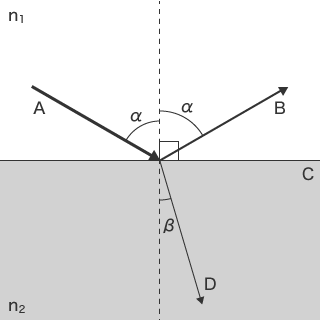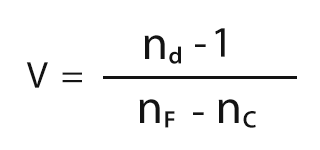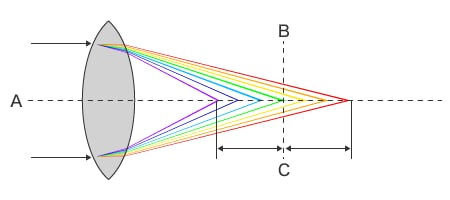Lenses are widely used optical parts in daily life found in various products such as cameras, microscopes, telescopes, and glasses. Lenses are roughly divided into two types: convex lenses and concave lenses. Optical lenses, such as telephoto lenses and zoom lenses, are created by combining multiple convex and concave lenses. This section explains the mechanism of lenses and introduces examples of their observation and measurement using digital microscopes.

## What is Refractive Index?

A refractive index is expressed with a value derived by dividing the speed of light in air by the speed of light in a substance.
As the speed of light varies according to the substance and the wavelength of light, the refractive index also varies according to the substance and wavelength of light. The direction in which light deflects can be calculated using Snell's law.

Snell's law (n1sinα = n2sinβ)

Refractive index 1 (e.g., air): n1
Refractive index 2 (e.g., water, glass): n2
Incident angle: α
Refraction angle: βA: Incident light B: Reflected light C: Reflecting surface D: Refracted light

## What is Dispersion?

When white light passes through a prism, the spectrum of light appears.
This phenomenon is called the dispersion of light. It occurs because the refractive index differs according to the wavelength of light.

The dispersion of an optical glass is expressed with a value called the Abbe number (v).Note: nd is the refractive index of the D line emitted by sodium atoms.

## Principle and Correction of Chromatic Aberration

As shown in the following figure, the focal position of light with short wavelengths is close to the lens and that of light with long wavelengths is further away from the lens due to the dispersion of light. When light with a specific wavelength is brought into focus, light with other wavelengths becomes out of focus, which blurs the colours of the image. This phenomenon is known as chromatic aberration.A: Light beam B: Focal point C: Differences in the focal distance

### What is spherical aberration?

Chromatic aberration occurs due to differences in the wavelengths of light. Aberration, however, occurs even with a single colour, called monochromatic aberration. A typical example is spherical aberration. A convex lens surface is in the shape of part of a sphere. Therefore, the closer a point is to the edge of a lens, the larger the incident angle, which then increases the refraction angle. The phenomenon in which the focal position differs between points close to the lens centre and points further away from the lens centre is called spherical aberration.

### How to correct spherical aberration

Spherical aberration can be offset by, for example, combining a convex lens with a concave lens, which has aberration in the opposite direction, or combining lenses that have different refractive indices.

Another method to offset spherical aberration is to use aspheric lenses. The spherical surface at the lens edge is processed to be a curved surface, instead of combining multiple lenses, which can correct the focal position without increasing the number of lenses.

## Fluorite (Calcium Fluoride, CaF2) Lenses that Minimise Chromatic Aberration

Fluorite lenses, which are made from fluorite, are used when chromatic aberration needs to be minimised.
Made from natural fluorite, fluorite lenses have a low refractive index and low dispersion properties, which cannot be found in ordinary types of glass.
Additionally, fluorite lenses have low dispersion properties for wavelengths of light from red to green and extraordinary partial dispersion properties that greatly disperse wavelengths of light from green to blue. KEYENCE’s digital microscopes use fluorite lenses, so they provide excellent images with low aberration.